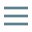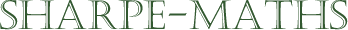Current topic:
Multiples & Factors
Next topic:
Primes

### Multiples

A multiple of a number is a number which occurs in that number's times table.

eg. multiples of 3 are 3, 6, 9, 12, 15...

Note that all numbers have an infinite number of multiples which are all bigger than or equal to the number itself.

Also note that 3 is the first multiple of 3 as it is the answer to the multiplication 3 × 1.

### Factors

Factors are numbers which can be divided exactly, into a number. This means that factors are always equal to or smaller than the number itself.

The factors of 6 are all of the numbers which will divide into 6 exactly, without leaving a remainder.

So the factors of 6 are 1, 2, 3, 6.

Please have a look at the Worked examples tab, above, for more details on finding factors and multiples and related topics.